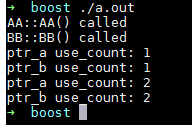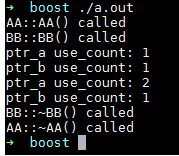## 智能指针shared_ptr新特性shared_from_this及weak_ptr 转

o
osc_962c7vo6

enable_shared_from_this是一个模板类，定义于头文件<memory>，其原型为：

template< class T > class enable_shared_from_this;
std::enable_shared_from_this 能让一个对象（假设其名为 t ，且已被一个 std::shared_ptr 对象 pt 管理）安全地生成其他额外的 std::shared_ptr 实例（假设名为 pt1, pt2, ... ） ，它们与 pt 共享对象 t 的所有权。
若一个类 T 继承 std::enable_shared_from_this<T> ，则会为该类 T 提供成员函数： shared_from_this 。 当 T 类型对象 t 被一个为名为 pt 的 std::shared_ptr<T> 类对象管理时，调用 T::shared_from_this 成员函数，将会返回一个新的 std::shared_ptr<T> 对象，它与 pt 共享 t 的所有权。

当类A被share_ptr管理，且在类A的成员函数里需要把当前类对象作为参数传给其他函数时，就需要传递一个指向自身的share_ptr。

1.为何不直接传递this指针

使用智能指针的初衷就是为了方便资源管理，如果在某些地方使用智能指针，某些地方使用原始指针，很容易破坏智能指针的语义，从而产生各种错误。

2.可以直接传递share_ptr<this>么？

答案是不能，因为这样会造成2个非共享的share_ptr指向同一个对象，未增加引用计数导对象被析构两次。例如：

#include <memory>
#include <iostream>

{
public:
}
};

int main()
{
// 错误的示例，每个shared_ptr都认为自己是对象仅有的所有者
// 打印bp1和bp2的引用计数
std::cout << "bp1.use_count() = " << bp1.use_count() << std::endl;
std::cout << "bp2.use_count() = " << bp2.use_count() << std::endl;

#include <memory>
#include <iostream>

struct Good : std::enable_shared_from_this<Good> // 注意：继承
{
public:
std::shared_ptr<Good> getptr() {
return shared_from_this();
}
~Good() { std::cout << "Good::~Good() called" << std::endl; }
};

int main()
{
// 大括号用于限制作用域，这样智能指针就能在system("pause")之前析构
{
std::shared_ptr<Good> gp1(new Good());
std::shared_ptr<Good> gp2 = gp1->getptr();
// 打印gp1和gp2的引用计数
std::cout << "gp1.use_count() = " << gp1.use_count() << std::endl;
std::cout << "gp2.use_count() = " << gp2.use_count() << std::endl;
}
system("pause");
}

因为在异步调用中，存在一个保活机制，异步函数执行的时间点我们是无法确定的，然而异步函数可能会使用到异步调用之前就存在的变量。为了保证该变量在异步函数执期间一直有效，我们可以传递一个指向自身的share_ptr给异步函数，这样在异步函数执行期间share_ptr所管理的对象就不会析构，所使用的变量也会一直有效了（保活）。

## C++智能指针 weak_ptr

weak_ptr 是一种不控制对象生命周期的智能指针, 它指向一个 shared_ptr 管理的对象. 进行该对象的内存管理的是那个强引用的 shared_ptr. weak_ptr只是提供了对管理对象的一个访问手段.
weak_ptr 设计的目的是为配合 shared_ptr 而引入的一种智能指针来协助 shared_ptr 工作, 它只可以从一个 shared_ptr 或另一个 weak_ptr 对象构造, 它的构造和析构不会引起引用记数的增加或减少.
定义在 memory 文件中(非memory.h), 命名空间为 std.

weak_ptr 使用:

std::shared_ptr<int> sp(new int(10));
std::weak_ptr<int> wp(sp);
wp = sp;
printf("%d\n", wp.use_count()); // 1
wp.reset();
printf("%d\n", wp); // 0

// 检查 weak_ptr 内部对象的合法性.
if (std::shared_ptr<int> sp = wp.lock())
{
}

### 成员函数

weak_ptr 没有重载*和->但可以使用 lock 获得一个可用的 shared_ptr 对象. 注意, weak_ptr 在使用前需要检查合法性.

expired 用于检测所管理的对象是否已经释放, 如果已经释放, 返回 true; 否则返回 false.
lock 用于获取所管理的对象的强引用(shared_ptr). 如果 expired 为 true, 返回一个空的 shared_ptr; 否则返回一个 shared_ptr, 其内部对象指向与 weak_ptr 相同.
use_count 返回与 shared_ptr 共享的对象的引用计数.
reset 将 weak_ptr 置空.
weak_ptr 支持拷贝或赋值, 但不会影响对应的 shared_ptr 内部对象的计数.

### 使用 weak_ptr 解决 shared_ptr 因循环引有不能释放资源的问题

对于弱引用来说, 当引用的对象活着的时候弱引用不一定存在. 仅仅是当它存在的时候的一个引用, 弱引用并不修改该对象的引用计数, 这意味这弱引用它并不对对象的内存进行管理.
weak_ptr 在功能上类似于普通指针, 然而一个比较大的区别是, 弱引用能检测到所管理的对象是否已经被释放, 从而避免访问非法内存。

``````#include <iostream>
#include <boost/smart_ptr.hpp>
using namespace std;
using namespace boost;

class BB;
class AA
{
public:
AA() { cout << "AA::AA() called" << endl; }
~AA() { cout << "AA::~AA() called" << endl; }
shared_ptr<BB> m_bb_ptr;  //!
};

class BB
{
public:
BB() { cout << "BB::BB() called" << endl; }
~BB() { cout << "BB::~BB() called" << endl; }
shared_ptr<AA> m_aa_ptr; //!
};

int main()
{
shared_ptr<AA> ptr_a (new AA);
shared_ptr<BB> ptr_b ( new BB);
cout << "ptr_a use_count: " << ptr_a.use_count() << endl;
cout << "ptr_b use_count: " << ptr_b.use_count() << endl;
//下面两句导致了AA与BB的循环引用，结果就是AA和BB对象都不会析构
ptr_a->m_bb_ptr = ptr_b;
ptr_b->m_aa_ptr = ptr_a;
cout << "ptr_a use_count: " << ptr_a.use_count() << endl;
cout << "ptr_b use_count: " << ptr_b.use_count() << endl;
}````````````#include <iostream>
#include <boost/smart_ptr.hpp>
using namespace std;
using namespace boost;

class BB;
class AA
{
public:
AA() { cout << "AA::AA() called" << endl; }
~AA() { cout << "AA::~AA() called" << endl; }
weak_ptr<BB> m_bb_ptr;  //!
};

class BB
{
public:
BB() { cout << "BB::BB() called" << endl; }
~BB() { cout << "BB::~BB() called" << endl; }
shared_ptr<AA> m_aa_ptr; //!
};

int main()
{
shared_ptr<AA> ptr_a (new AA);
shared_ptr<BB> ptr_b ( new BB);
cout << "ptr_a use_count: " << ptr_a.use_count() << endl;
cout << "ptr_b use_count: " << ptr_b.use_count() << endl;
//下面两句导致了AA与BB的循环引用，结果就是AA和BB对象都不会析构
ptr_a->m_bb_ptr = ptr_b;
ptr_b->m_aa_ptr = ptr_a;
cout << "ptr_a use_count: " << ptr_a.use_count() << endl;
cout << "ptr_b use_count: " << ptr_b.use_count() << endl;
}``````因此，如果代码比较复杂，我们在使用shared_ptr的时候其实很难知道是否会有循环引用，所以即使有weak_ptr来解决这个问题，我们也不太容易知道何时能用到，除非清楚非常清楚类之间的关系，所以，在我们编程的时候尽量使用一个指针控制生命周期（即使用shared_ptr），而多个指针控制访问（即使用weak_ptr）。

o

### osc_962c7vo6

Netty那点事（三）Channel与Pipeline

Channel是理解和使用Netty的核心。Channel的涉及内容较多，这里我使用由浅入深的介绍方法。在这篇文章中，我们主要介绍Channel部分中Pipeline实现机制。为了避免枯燥，借用一下《盗梦空间》的...

2013/11/24
2W
22
【opencv】图形的绘制

1.矩形图像的绘制： 原函数：void cvRectangle(CvArr* img, CvPoint pt1, CvPoint pt2, CvScalar color, int thickness=1, int line_type=8,int shift=0) img就是需要绘制的图像 pt1 and pt......

2014/10/08
1.2K
1
Swift百万线程攻破单例（Singleton)模式

2014/06/20
3.5K
16

2013/11/09
7.7K
8

Castle Game Engine 是一个用 Object Pascal 开发的跨平台 3D 游戏引擎。包含一个灵活的 3D 对象系统与开箱即用的水平,项目,智能生物等等。使用 X3D、VRML、Collada 和其他格式实现渲染和处理...

2013/02/05
2.1K
0

DSJ-shitou
39分钟前
8
0
OSChina 周五乱弹 —— 特么是别的公司派来的特洛伊木马吧？

Osc乱弹歌单（2020）请戳（这里） 【今日歌曲】 小小编辑推荐：《我会守在这里》- 毛不易 《我会守在这里》- 毛不易 手机党少年们想听歌，请使劲儿戳（这里） @FalconChen ：股市连跪了五天，...

40分钟前
48
2

73
0
《Java8实战》笔记（02）：通过行为参数传递代码

19
0
JeeSite 4 架构特点、安全方面、为什么好、工匠精神、不忘初心

1、底层架构 以 Spring Boot 2 为基础，Maven 多项目依赖，模块分项目，松耦合，方便模块升级、增减模块。 模块化的数据库自动升级程序，当模块升级代码需要更新数据库时，自动执行对应版本 ...

ThinkGem

13
0